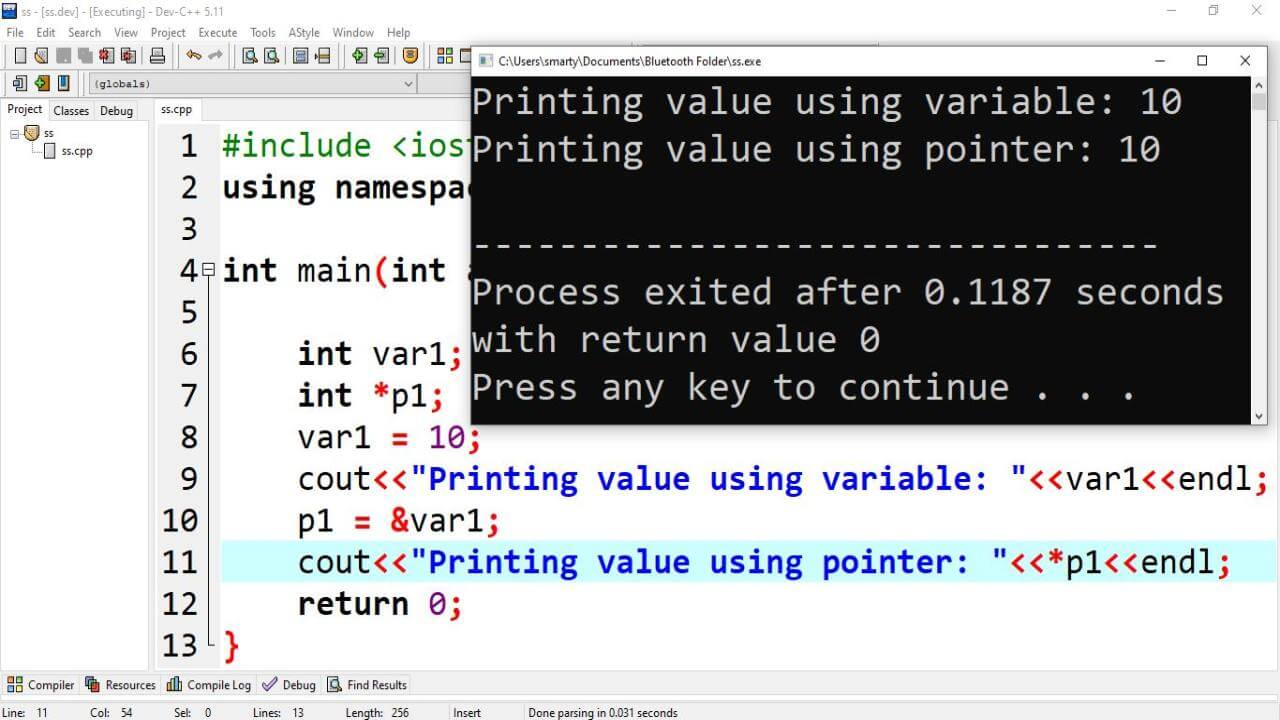# Pointers in C++

Pointers are variables that are used to store address of memory locations. Similar to variables pointers also can have multiple types. Address of variable are stored in similar type pointers. Such as if we want to store address of int type variable then we have to declare pointer of int type. Pointers and variable can also be declared in a single declaration statement in C and C++.

## Pointer Declation Syntax:

Pointers are declared in the same way as a normal variable is declared means using data type and valid identifier name. Pointer declaration also ends with semi colon. We can declare multiple pointers in a single declaration statement. We have to write dereference operator with identifier.

``````int *p1, *p2;
// or simply
int* p1,p1;``````

## C++ Pointer Uses:

Pointers are used for the following purposes.

• Access memory locations
• Create dynamic memory locations
• Accessing array elements

This statement is used to assign address of variable to pointer.

p = &a; // where p is pointer and a is variable. Both are of same type.

## C++ Pointer Example:

C++ program that demonstrate the fundamental use of pointer is as provided below.

``````#include <iostream>
using namespace std;

int main(int argc, char** argv) {

int var1;
int *p1;
var1 = 10;
cout<<"Printing value using variable: "<<var1<<endl;
p1 = &var1;
cout<<"Printing value using pointer: "<<*p1<<endl;
return 0;
}``````

### Example Output:## Types of Pointers:

There are commonly used four types of pointers in C++.

### 1. NULL Pointer:

A pointer variable that is initialized by NULL is called null pointer. It is good practice to initialize all pointers at the time of declaration.

#### Example of Null Pointer:

#include <iostream>
using namespace std;

int main(int argc, char** argv) {
int *ptr = NULL;
cout<<"Value of NULL pointer is "<<ptr<<endl;
cout<<"Size of NULL pointer is "<<sizeof(ptr)<<endl;
return 0;
}

#### Output:

``````Value of NULL pointer is 0
Size of NULL pointer is 8``````

### 2. Generic Pointer:

Generic pointer is also called void/ dynamic pointer. Data type of generic pointer is void. It can hold address of any data type variable. Size of void pointer depend on compiler being used. Normally it occupy 8 bytes in memory.

#### Example of Void Pointer:

#include <iostream>
using namespace std;

int main(int argc, char** argv) {
int var1 = 10;
void *ptr = &var1;
cout<<"Value Using Void pointer: "<<*static_cast<int*>(ptr)<<endl;
cout<<"Size of void pointer is "<<sizeof(var1)<<endl;
return 0;
}

``````Value Using Void pointer: 10
Size of void pointer is 4``````

### 3. Wild Pointer:

It is considered as most critical type of pointer in C++. Any pointer that is not properly initialized is called wild pointer. Wild pointer may point to an un authorized memory lcation which may cause program crash and loss of data.

#### Example of Wild Pointer:

#include <iostream>
using namespace std;

int main(int argc, char** argv) {
float *ptr;
cout<<"Value of Wild pointer: "<<ptr<<endl;
cout<<"Size of Wild pointer: "<<sizeof(ptr)<<endl;
return 0;
}

#### Output:

In output 0x1 is address of unknown memory location.

``````Value of Wild pointer: 0x1
Size of Wild pointer: 8``````